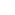Part of the Oxford Instruments Group
Expand

# Visualize Surface CurvatureThis function evaluates curvature of a surface by making spots at each vertex and calculating curvature of these spots with respect to their neighbors. The function was developed in collaboration with the Bitplane technical support team and incorporates the Patch Curvature algorithm developed by Dirk-Jan Kroon in MATLAB code. This script was written to evaluate 3D surface curvature of the mouse uterine lumen at preimplantation and implantation stages of pregnancy. 3D curvature measurements were generated by modifying an existing script intended to calculate localized, small area, curvature. The script was modified to calculate the curvature in larger objects, such as the uterus. The analysis was carried out using the vertices generated in the Surface mode in Imaris. Surface complexity is reduced by evenly reducing the number of vertices to one-tenth of the original number. Using the normals in each axis at each vertex, the radius of the curve formed by connecting neighbor vertices was calculated. Radii calculated with six neighbor vertices are averaged to obtain curvature mean and multiplied by 10 to calculate Cmean. Cmean of the surface can then be displayed as a heat map. The script also generates a bar graph with total number of vertices within Cmean 0-0.1, 0.1-0.15, 0.15-0.45 and 0.45-0.675. On the bar graph the script calculates a value for folding factor (f), which is the ratio of the Cmean for the highly curved surface (0.1250.3), to the least curved surface (00.05). Morphology of uterine luminal segments is thus expressed as the folding factor ( f ). The script was created in Imaris version 8.1.2 and has been tested in Imaris version 8.4. This script was a part of the publication "Insights from imaging the implanting embryo and the uterine environment in three dimensions", Arora et al (Development, 2016, PMID: 27836961).

Date: Dec 2016

Author: Ripla Arora

Category: File XTension